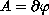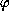Electron. J. Diff. Eqns. Monograph 03, 2002.

### Periodic solutions for evolution equations Mihai Bostan

Abstract:
We study the existence and uniqueness of periodic solutions for evolution equations. First we analyze the one-dimensional case. Then for arbitrary dimensions (finite or not), we consider linear symmetric operators. We also prove the same results for non-linear sub-differential operatorswhereis convex.

Submitted May 14, 2002. Published August 23, 2002.
Math Subject Classifications: 34B05, 34G10, 34G20.
Key Words: maximal monotone operators, evolution equations, Hille-Yosida's theory.

Show me the PDF file (351K), TEX file, and other files for this article.Mihai Bostan Universite de Franche-Comte 16 route de Gray F-25030 Besancon Cedex, France mbostan@math.univ-fcomte.fr